# CBSE Solutions for Class 10 English

#### GSEB std 10 science solution for Gujarati check Subject Chapters Wise::

In a simultaneous toss of two coins, find the probability of getting exactly one head,

જવાબ : ​​​​​​The sample space is given by
S = {HH, HT, TH, TT}
Total events = 4
exactly one head = {HT, TH} = 2
P(one head) = 2/4 = 1/2

An unbiased die is thrown. What is the probability of getting an odd number or a multiple of 3?

જવાબ : 2/3

Maximum and minimum value of probability is

જવાબ : ​​​​​(1,0)

If E be any event, then value of P(E)lie in between

જવાબ : 0≤P(E)≤1

A dice is thrown once, what is the probability of getting a prime number?

જવાબ :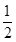One card is drawn from a well shuffled deck of 52 cards. Find the probability of drawing ‘10’ of a red suit.

જવાબ : 1/26

What is the probability of getting number not more than 7 in a single throw of die

જવાબ : 1

The king, queen and jack of clubs are removed from a deck of 52 playing cards and then well shuffled. One card is selected from the remaining cards, find the probability of getting a queen.

જવાબ :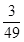Cards marked with the numbers 1 to 50 are placed in a box and mixed throughly. One card is drawn from this box, find the probability that the number on the card is an even number.

જવાબ :What is the probability that a number selected from the numbers 1,2,3,…15 can be divisible by 4?

જવાબ :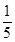A dice is thrown once, what is the probability of getting a prime number?

જવાબ :A bag contains 5 blue and 4 purple balls. A ball is drawn at random from the bag. What is the probability of getting a purple ball?

જવાબ : 4/9

Cards each marked with one of the numbers 4,5,6,…20 are placed in a bag and mixed thoroughly. One card is drawn at random from the bag, what is the probability of getting an even prime number?

જવાબ : 0

12 defective pencils are accidently mixed with 132 good ones. It is not possible to just look at a pen and tell whether or not it is defective. One pen is taken out at random from this lot. Determine the probability that the pen taken out is a good one.

જવાબ :

Total number of favourable outcomes = 132 + 12 = 144 Number of favourable outcomes = 132

Hence, P (getting a good pencil) =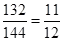It is given that in a group of 3 students, the probability of 2 students not having the same birthday is 0.99. What is the probability that the 2 students have the same birthday?

જવાબ :

Let E be the event of having the same birthday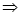P(E) = 0.99

But P(E) + P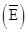= 1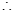P= 1 P(E) = 1 0.99 = 0.01

If P(E) = 0.04, what is the probability of ‘not E’?

જવાબ :

Since P(E) + P (not E) = 1P (not E) = 1 – P(E) = 1 – 0.04 = 0.96

The probability of an event is greater than or equal to                         and less than or equal to                           .

જવાબ : 0,1

The sum of the probabilities of all the elementary events of an experiment is

.

જવાબ : 1

The probability of an event that is certain to happen is                       . Such an event is called

જવાબ : 1, Certain Event

The probability of an event that cannot happen is                      . Such an event is called                           .

જવાબ : 0, impossible event

Probability of event E + Probability of event “not E” =

જવાબ : 1

In a simultaneous toss of two coins, find the probability of getting atmost one head. (2012D)

જવાબ : The sample space is given by
S = {HH, HT, TH, TT}
Total events = 4

atmost one head = {HT, TH, TT} = 3
P(atmost one head) = ¾

Two coins are tossed simultaneously. Find the probability of getting at least one head. (2013OD)

જવાબ : S = {HH, HT, TH, TT}, i.e., 4
P (atleast one head) = 3/4

Three coins are tossed simultaneously. Find the probability of getting exactly two heads. (2013OD)

જવાબ : S = {HHH, HHT, HTH, THH, TTH, THT, HTT, TTT} = 8
P(exactly two heads) = 3/8

Rahim tosses two different coins simultaneously. Find the probability of getting at least one tail. (2014D)

જવાબ : S = {HH, HT, TH, TT}, i.e., 3
P(at least one tail) = ¾

A card is drawn at random from a pack of 52 playing cards. Find the probability that the card drawn is neither an ace nor a king. (2011OD).

જવાબ : P (neither an ace nor a king)
= 1 – P (either an ace or a king)
= 1 – [P (an ace) + P (a king)]A card is drawn at random from a well shuffled pack of 52 playing cards. Find the probability that the drawn card is neither a king nor a queen. (2013D)

જવાબ : P (neither a king nor a queen)
= 1 – P (king or queen)A card is drawn at random from a well shuffled pack of 52 playing cards. Find the probability that the drawn card is neither a jack nor an ace. (2013D)

જવાબ : Total number of cards = 52
Numbers of jacks = 4
Numbers of aces = 4
Card is neither a jack nor an ace
= 52 – 4 – 4 = 44

Required probability = 44/52 = 11/13

Two dice are thrown simultaneously. Find the probability of getting a doublet. (2013OD)

જવાબ : Two dice can be thrown as 6 x 6 = 36 ways
“a doublet” can be obtained by (1, 1), (2, 2), (3, 3), (4, 4), (5, 5), (6, 6), i.e., 6 ways
P(a doublet) = 6/3}= 1/6

A dice is tossed once. Find the probability of getting an even number or a multiple of 3. (2013D)

જવાબ : S = {1, 2, 3, 4, 5, 6} = 6
‘an even number or multiple of 3’ are 2, 3, 4, 6, i.e., 4 numbers

Required probability = 4/6 = 2/3

Two different dice are tossed together. Find the probability that the number on each die is even.

જવાબ : Two dice can be thrown as 6 × 6 = 36 ways
The probability of number on each die is even areTwo different dice are tossed together. Find the probability that the sum of numbers appearing on the two dice is 5.

જવાબ : Two dice can be thrown as 6 × 6 = 36 waysA coin is tossed two times. Find the probability of getting at least one head. (2011D)

જવાબ : S = {HH, HT, TH, TT}
Total number of ways = 4
Atleast one head = {HH, HT, TH}, i.e., 3 ways

P (atleast one head) =3/4

A coin is tossed two times. Find the probability of getting not more than one head. (2011D)

જવાબ : S = {HH, HT, TH, TT} = 4
Favourable cases are HT, TH, TT i.e., 3 cases

P (not more than 1 head) = 3/4

Three distinct coins are tossed together. Find the probability of getting at least 2 heads.

જવાબ : Total number of possible outcomes = 21 = 23 = 8
(HHH, TIT, HHT, THH, THT, HTH, TTH, HTT)
Possible outcomes of at least two heads = 4
(HHT, THH, HHH, HTH)

P(at least two heads) = 4/8 =1/2

Three distinct coins are tossed together. Find the probability of getting at most 2 heads.

જવાબ : Total number of possible outcomes = 21 = 23 = 8
(HHH, TIT, HHT, THH, THT, HTH, TTH, HTT)
Possible outcomes of at most two heads = 7
(HHT, TTT, THH, THT, HTH, TTH, HTT)

P(at most two heads) = 7/8

Three different coins are tossed together. Find the probability of getting exactly two heads.

જવાબ : S {HHH, HHT, HTH, THH, TTH, THT, HTT, TTT}
Total number of ways = 8
(i) Exactly two heads
= HHT, HTH, THH, i.e., 3 ways

P (exactly two heads) = 3/8

Three different coins are tossed together. Find the probability of getting at least two heads.

જવાબ : S {HHH, HHT, HTH, THH, TTH, THT, HTT, TTT}
Total number of ways = 8
Atleast two heads= HHT, HTH, THH, HHH i.e., 4 ways

P (atleast two heads) = 4/8=1/2

Three different coins are tossed together. Find the probability of getting at least two tails.

જવાબ : S {HHH, HHT, HTH, THH, TTH, THT, HTT, TTT}
Total number of ways = 8
Atleast two tails= TTH, THT, HTT,TTT i.e., 4 ways

P (atleast two tails) = 4/8=1/2

A coin is tossed two times. Find the probability of getting both heads or both tails. (2011D)

જવાબ : S = {HH, HT, TH, TT) = 4
P (both head or both tail)
= P (both heads) + P (both tails)
= 1/4+ ¼ = 1/2

An unbiased die is thrown. What is the probability of getting an odd number or a multiple of 3?

જવાબ : 2/3

Maximum and minimum value of probability is                          .

જવાબ : ​​​​​(1,0)

If E be any event, then value of P(E)lie in between

જવાબ : ​​​​​0≤P(E)≤1

A dice is thrown once, what is the probability of getting a prime number?

જવાબ : 1/2

One card is drawn from a well shuffled deck of 52 cards. Find the probability of drawing ‘10’ of a red suit.

જવાબ : 1/26

What is the probability of getting number not more than 7 in a single throw of die.

જવાબ : 1

The king, queen and jack of clubs are removed from a deck of 52 playing cards and then well shuffled. One card is selected from the remaining cards, find the probability of getting a queen.

જવાબ : 3/49

Cards marked with the numbers 1 to 50 are placed in a box and mixed throughly. One card is drawn from this box, find the probability that the number on the card is an even number.

જવાબ : 1/2

What is the probability that a number selected from the numbers 1,2,3,…15 can be divisible by 4?

જવાબ : 1/5

A dice is thrown once, what is the probability of getting a prime number?

જવાબ : 1/2

One card is drawn from a well-shuffled deck of 52 cards. Find the probability of getting:

(i)a king of black colour

(ii)a face card

(iii)a black face card

(iv)the queen of hearts

(vi)the queen of diamonds.

જવાબ :

Each suit contains one king.

Favourable outcomes = 1

Hence, P (a king of black colour) =2/52 =1/26

(ii)There are 12 face cards in a pack.

Favourable outcomes = 12

Hence, P (a face card) =12/52 = 3/13

(iii)There are two suits of black cards, i.e., spade and club. Each suit contains 3 face cards.

Favourable outcomes = 2 x 3 = 6

Hence, P (a black face card) = 6/52 = 3/26

(iv)There are only one queen of heart.

Favourable outcome = 1

Hence, P (the quen of hearts) =1/52

(v)There are 13 cards of spade.

Favourable outcomes = 13

Hence, P (a spade) =13/52 = 1/4

(vi)There is only one queen of diamonds.

Favourable outcome = 1

Hence, P (the queen of diamonds) =1/52

​​​​​Two customers Shyam and Ram are visiting a particular shop in the same week (Tuesday to Saturday). Each is equally likely to visit the shop on any day as on another day. What is the probability that both will visit the shop on

(i) the same day?

(ii) consecutive days?

(iii) different days?

જવાબ :

Total favourable outcomes associated to the random experiment of visiting a particular shop in the same week (Tuesday to Saturday) by two customers Shyam and Ram are:

(T, T) (T, W) (T, TH) (T, F) (T, S)

(W, T) (W, W) (W, TH) (W, F) (W, S)

(TH, T) (TH, W) (TH, TH) (TH, F) (TH, S)

(F, T) (F, W) (F, TH) (F, F) (F, S)

(S, T) (S, W) (S, TH) (S, F) (S< S)Total number of favourable outcomes = 25

1. The favourable outcomes of visiting on the same day are (T, T), (W, W), (TH, TH), (F, F) and (S, S).Number of favourable outcomes = 5

Hence required probability = 5/25 =1/5

1. The favourable outcomes of visiting on consecutive days are (T, W), (W, T), (W, TH), (TH, W), (TH, F), (F, TH), (S, F) and (F, S).Number of favourable outcomes = 8

Hence required probability =8/25

1. Number of favourable outcomes of visiting on different days are 25 – 5 = 20Number of favourable outcomes = 20

Hence required probability =20/25=4/5

A card is drawn at random from a well shuffled deck of playing cards. Find the probability that the card drawn is
(i)a card of spades of 10
(ii)a red Jack
(iii)neither a king nor a queen
(iv)either a king or a queen
(v)a face card
(vi)cards which is neither king nor a red card.

જવાબ :

Total possible outcomes = 52

(i)No. of spades = 13 No. of ‘10’ card = 4
1 card is common [10 of spade] Favourable outcomes = 13+4 – 1=16
Required probability = 16/52 =4/13

(ii)No. of red jacks = 2 Favourable outcomes = 2
Required probability = 2/52 =1/26

(iii)No. of king and queen = 4+4=8
Favourable outcomes = 52 – 8 = 44
Required probability = 44/52 =11/13

(iv)No. of king and queen =4+4=8 Required probability = 8/52 =2/13

(v)No. of face cards = 4+4+4=12 [Jack, queen and king are face card]

Required probability =12/52=3/13

(vi)No. of cards which are neither red card nor king = 52 – (26+4 – 2)=52 – 28=24

Required probability =24/52 =6/13

Cards marked with numbers 1,2,3,…25 are placed in a bag and mixed thoroughly and one card is drawn at random from the bag, what is the probability that the number on the card is
(i)    a prime number?
(ii)    a multiple of 3 or 5?
(iii)    an odd number?
(iv)    neither divisible by 5 nor by 10?
(v)    perfect square?
(vi)    a two-digit number?

જવાબ : Total no. of possible outcomes = 25

(i)favourable cases are 2,3,5,7,11,13,17,19,23 which are 9 in number Required probability =  9/25

(ii)Multiple of 3 or 5
Favourable cases are 3,5,6,9,10,12,15,18,20,21,24,25, which are 12 in number
Required probability =12/25

(iii)Favourable cases are 1,3,5,7,9,11,13,15,17,19,21,23,25, which are 13 in number Required probability =13/25

(iv)Favourable cases are 1,2,3,4,6,7,8,9,11,12,13,14,16,17,18,19,21,22,23,24, which are 20 in number
Required probability = 20/25 = 4/5

(v)Perfect square numbers are 1,4,9,16,25 Favourable cases are = 5
Required probability = 5/25 = 1/5

(vi)Two-digit numbers are 10,11,12,13,14,15,16,17,18,19,20,21,22,23,24,25 =16
Required probability =16/25

All the black face cards are removed from a pack of 52 playing cards. The remaining cards are well shuffled and then a card is drawn at random. Find the probability of getting a:
(i) face card
(ii) red card
(iii) black card
(iv) king (2014D)

જવાબ : number of cards = 52
Black face cards = 6
Remaining cards = 52 – 6 = 46A card is drawn from a well shuffled deck of 52 cards. Find the probability of getting
(i) a king of red colour
(ii) a face card
(iii) the queen of diamonds. (2012 D)

જવાબ : (i) cards in a deck = 52
no. of kings = 4
no. of red kings = 2A number x is selected at random from the numbers 1, 4, 9, 16 and another number y is selected at random from the numbers 1, 2, 3, 4. Find the probability that the value of xy is more than 16. (2016OD)

જવાબ : x can be any one of 1, 4, 9, or 16, i.e., 4 ways
y can be any one of 1, 2, 3, or 4, i.e., 4 ways
Total number of cases of xy = 4 × 4 = 16 ways
Number of cases, where product is more than 16
(9, 2), (9, 3), (9, 4), (16, 2), (16, 3), (16, 4), i.e., 6 ways
Required probability = 6/16 = 3/8

A number x is selected at random from the numbers 1, 2, 3 and 4. Another number y is selected at random from the numbers 1, 4, 9 and 16. Find the probability that product of x and y is less than 16. (2016OD)

જવાબ : X can be any one of 1, 2, 3, and 4 i.e., 4 ways
Y can be any one of 1, 4, 9, and 16 i.e., 4 ways
Total no. of cases of XY = 4 × 4 = 16 ways
No. of cases, where product is less than 16 (1, 1), (1, 4), (1, 9), (2, 1), (2, 4), (3, 1), (3, 4), (4,1) i.e., 8 ways
P (product x & y less then 16) = 8/16 = 1/2

A game of chance consists of spinning an arrow on a 3 circular board, divided into / 4 8 equal parts, which comes to rest pointing at one of the numbers 1, 2, 3, …, 8, which are equally likely outcomes. What is the probability that the arrow will point at
(i) an odd number
(ii) a number greater than 3
(iii) a number less than 9. (2016 D)

જવાબ : Total numbers = 8
(i) “Odd numbers” are 1, 3, 5, 7, i.e., 4
P(an odd number) = 4/8 =1/2

(ii) “nos. greater than 3” are 4, 5, 6, 7, 8, i.e., 5
P(a number > 3) = 5/8

(iii) “numbers less than 9” are 1, 2, 3, …8 i.e., 8
P(a number < 9) = 8/8 = 1

A bag contains 25 cards numbered from 1 to 25. A card is drawn at random from the bag. Find the probability that the number on the drawn card is: (2015D)
(i) divisible by 3 or 5
(ii) a perfect square number.

જવાબ : Total number of outcomes = 25
(i) Possible outcomes of numbers divisible by 3 or 5 in numbers 1 to 25 are (3, 6, 9, 12, 15, 18, 21, 24, 5, 10, 20, 25) = 12
P(No. divisible by 3 or 5) = 12/25

(ii) Possible outcomes of numbers which are a perfect square = 5, i.e., (1, 4, 9, 16, 25)
P(a perfect square no.) = 5/25 = 1/5

A box contains 20 cards numbered from 1 to 20. A card is drawn at random from the box. Find the probability that the number on the drawn card is (2015D)
(i) divisible by 2 or 3,
(ii) a prime number.

જવાબ : (i) Numbers divisible by 2 or 3 from 1 to 20 are 2, 4, 6, 8, 10, 12, 14, 16, 18, 3, 9, 15, 20 = 13
Total outcomes = 20
Possible outcomes = 13
P(divisible by 2 or 3) = 13/20

(ii) Prime numbers from 1 to 20 are 2, 3, 5, 7, 11, 13, 17, 19 = 8
Total Outcomes = 20
Possible outcomes = 8
P(a prime number) = 8/20 = 2/5

A bag contains cards numbered from 1 to 49. A card is drawn from the bag at random, after mixing the cards thoroughly. Find the probability that the number on the drawn card is:
(i) an odd number
(ii) a multiple of 5
(iii) a perfect square
(iv) an even prime number (2014D)

જવાબ : Total number of cards = 49
(i) Odd numbers are 1, 3, 5, …., 49, i.e., 25
P(an odd number) = 25/49

(ii) ‘A multiple of 5’ numbers are 5, 10, 15, ……., 45, i.e., 9
P(a multiple of 5) = 9/49

(iii) “A perfect square” numbers are 1, 4, 9, …….., 49, i.e., 7
P(a perfect square number) = 7/49 = 1/7

(iv) “An even prime number” is 2, i.e., only one number
P(an even prime number) = 1/49

Find the probability that a leap year selected at random, will contain 53 Mondays. (2013OD)

જવાબ : In a leap year, total number of days = 366
366 days
= 52 complete weeks + 2 extra days
Thus, a leap year always has 52 Mondays and extra 2 days.
Extra 2 days can be,
(i) Sunday and Monday
(ii) Monday and Tuesday
(iii) Tuesday and Wedn
esday
(iv) Wednesday and Thursday
(v) Thursday and Friday
(vi) Friday and Saturday
(vii) Saturday and Sunday
Let E be the event that a leap year has 53 Mondays.
E = {Sun and Mon, Mon and Tues}
P(E) = 2/7

A box contains cards numbered 3, 5, 7, 9, …, 35, 37. A card is drawn at random from the box. Find the probability that the number on the drawn card is a prime number. (2013OD)

જવાબ : Total number of cards = 18
Prime numbers are: 3, 5, 7, 11, 13, 17, 19, 23, 29, 31, 37, i.e., 11
P(Prime number) = 11/180

A group consists of 12 persons, of which 3 are extremely patient, other 6 are extremely honest and rest are extremely kind. A person from the group is selected at random. Assuming that each person is equally likely to be selected, find the probability of selecting a person who is (2013D)
(i) extremely patient
(ii) extremely kind or honest.

જવાબ : Extremely patient = 3     …..[given in ques.
Extremely honest = 6
Extremely kind = 12 – 3 – 6 = 3A box contains 70 cards numbered from 1 to 70. If one card is drawn at random from the box, find the probability that it bears
(i) a perfect square number.
(ii) a number divisible by 2 and 3. (2012OD)

જવાબ : (i) Perfect squares till 70 are
12, 22, …, 82 = 8

P(a perfect square) = 8/70= 4/35
(ii) Number divisible by 2 and 3 till 70 are:
6, 12, 18, …, 66, i.e., 11
nos.
P(a no. divisible by 2 and 3) = 11/70

A box contains 35 blue, 25 white and 40 red ‘marbles. If a marble is drawn at random from the box, find the probability that the drawn marble is (i) white (ii) not blue (iii) neither white nor blue. (2012D)

જવાબ : Number of blue marbles = 35
Number of white marbles = 25
Number of red marbles = 40
Total Number of marbles = 35 + 25 + 40 = 100(iii)P(red) = 40/100 = 2/5

A survey has been done on 100 people out of which 20 use bicycles, 50 use motorbikes and 30 use cars to travel from one place to another. Find the probability of persons who use bicycles, motorbikes and cars respectively? (2011D)

જવાબ :

P(bicycles) = 20/100 = 0.2

P(motorbikes) = 50/100 = 0.5

P(cars) = 30/100 = 0.3

### There are No Content Availble For this Chapter

 1 Total outcomes for a dice A 2 2 Total outcomes for 2 dice B 6 3 Total outcomes for a coin C 4 4 Total outcomes for 2 coin D 36

જવાબ :

1-B, 2-D, 3-A, 4-C

Probability for 2 coins tossed at a time

 1 P(2 heads) A 4 2 P(Total outcomes) B 1/4 3 P(one head & one tail) C 3/4 4 P(atleast one head) D 1/2

જવાબ :

1-B, 2-A, 3-D, 4-C

Probability for 3 coins tossed at a time

 1 P(2 heads) A 1/2 2 P(atleast 2 tails) B 7/8 3 P(3 heads) C 3/8 4 P(atleast 1 tail) D 1/8

જવાબ :

1-C, 2-A, 3-D, 4-B

Probability for 1 dice rolled

 1 P(6) A 2/6 2 P(even) B 1/6 3 P(divisible of 3) C 4/6 4 P(>2) D 3/6

જવાબ :

1-B, 2-D, 3-A, 4-C

Probability for 2 dice rolled at a time

 1 P(2  three’s) A 16/36 2 P(atleast 2 two’s) B 4/36 3 P(total atleast six) C 11/36 4 P(total of 5) D 1/36

જવાબ :

1-D, 2-C, 3-A, 4-B

 1 Total outcomes for 3 dice A 8 2 Total outcomes for 4 dice B 196 3 Total outcomes for 3 coin C 16 4 Total outcomes for 4 coin D 1296

જવાબ :

1-B, 2-D, 3-A, 4-C

Probability for 2 dice rolled at a time

 1 P(2  TWO’s) A 16/36 2 P(atleast 2 SIX’s) B 3/36 3 P(total atleast six) C 11/36 4 P(total of 4) D 1/36

જવાબ :

1-D, 2-C, 3-A, 4-B

Probability for 1 dice rolled

 1 P(<6) A 1/6 2 P(odd prime no.) B 5/6 3 P(divisible of 2) C 2/6 4 P(2) D 3/6

જવાબ :

1-B, 2-C, 3-D, 4-A

Probability for 2 coins tossed at a time

 1 P(2 Tails) A 1 2 P(either head or tail) B 1/4 3 P(atleast one tails) C 0 4 P(neither head nor tail) D 3/2

જવાબ :

1-B, 2-A, 3-D, 4-C

Probability for 3 coins tossed at a time

 1 P(3 tails) A 4/8 2 P(less than 2 tails) B 7/8 3 P(1 tails) C 1/8 4 P(atleast 1 tails) D 3/8

જવાબ :

1-C, 2-A, 3-D, 4-B

### Take a Test

Choose your Test :

### Probability

Math
Chapter 15 : Probability

### Browse & Download CBSE Books For Class 10 All Subjects

The GSEB Books for class 10 are designed as per the syllabus followed Gujarat Secondary and Higher Secondary Education Board provides key detailed, and a through solutions to all the questions relating to the GSEB textbooks.

The purpose is to provide help to the students with their homework, preparing for the examinations and personal learning. These books are very helpful for the preparation of examination.

For more details about the GSEB books for Class 10, you can access the PDF which is as in the above given links for the same.